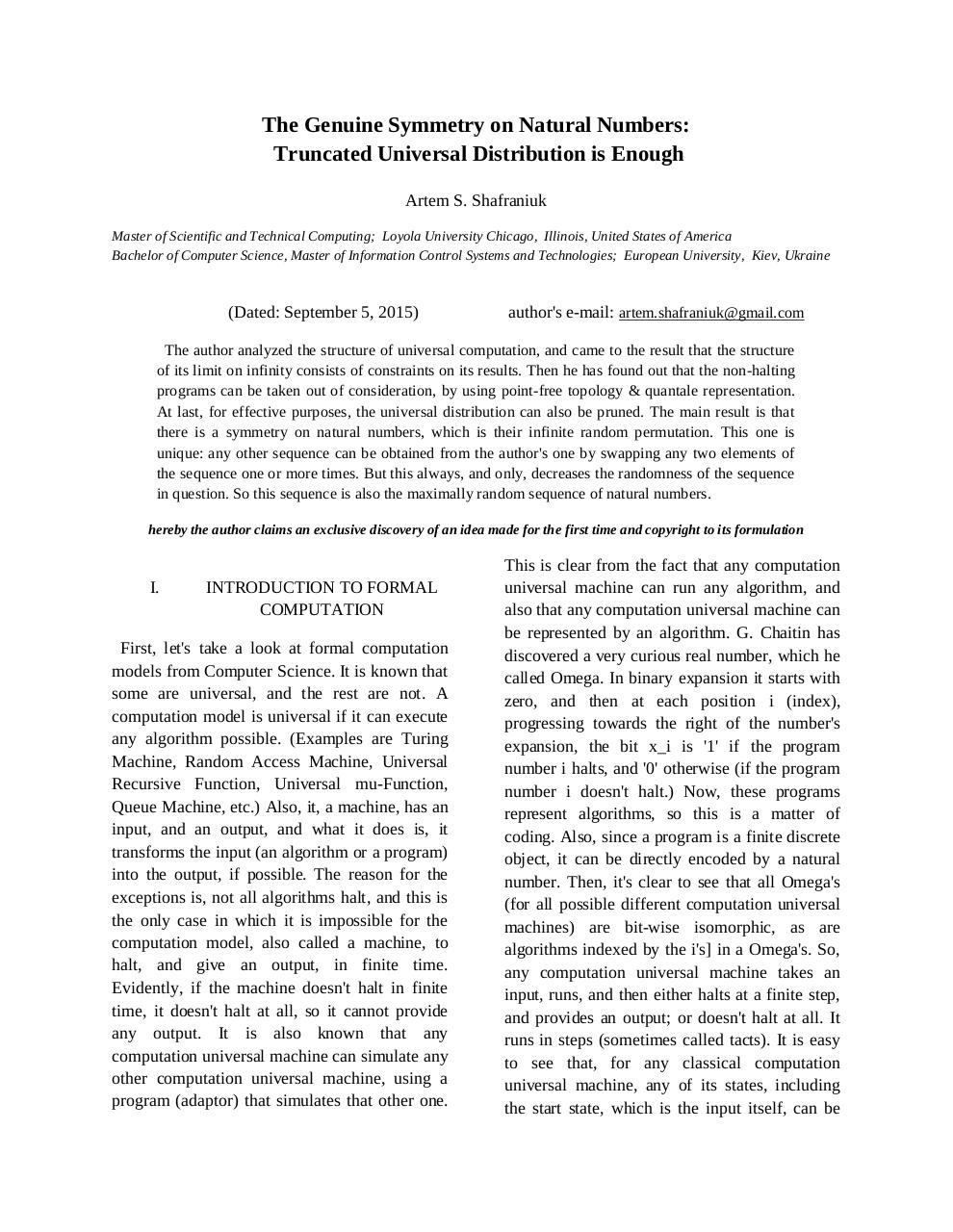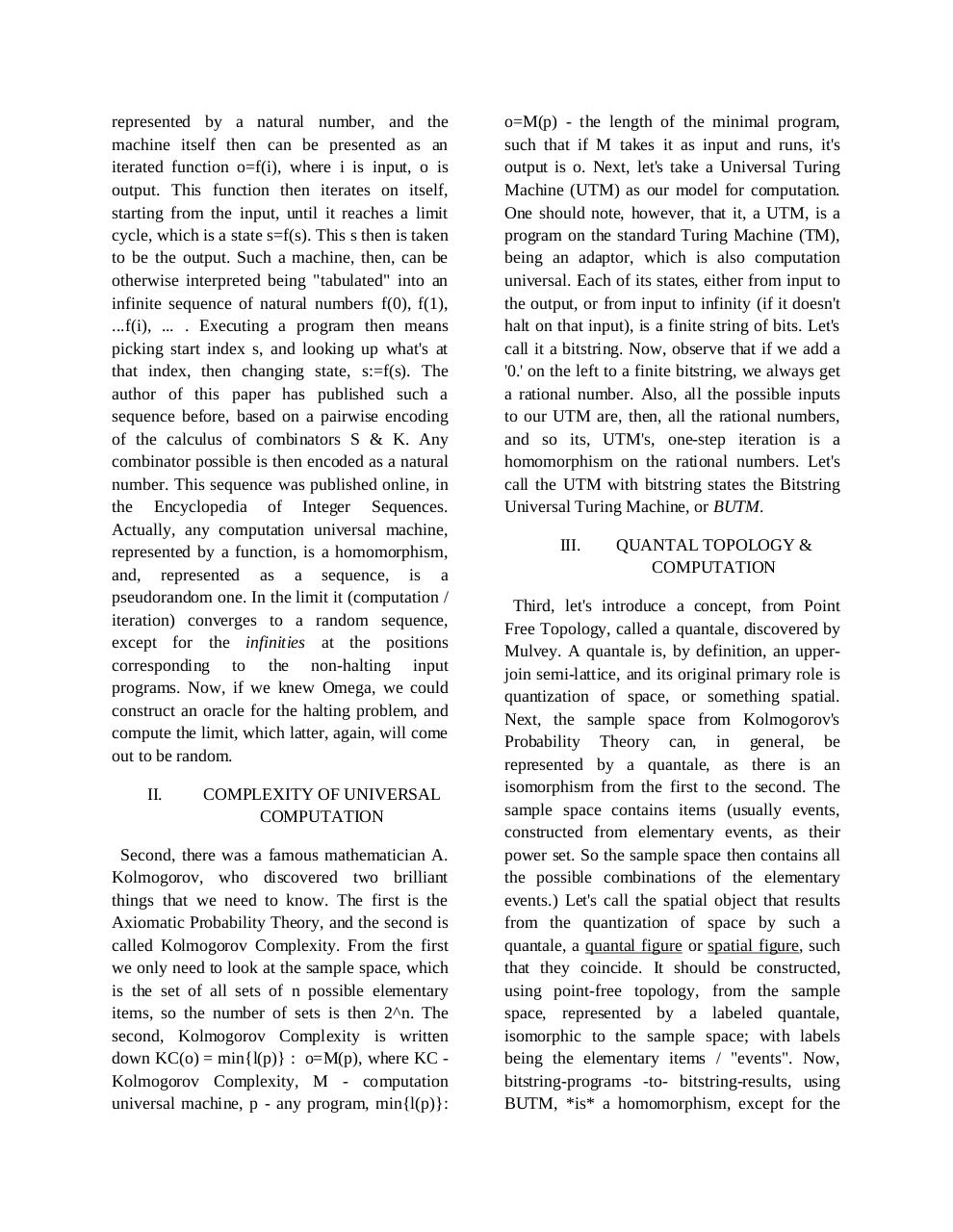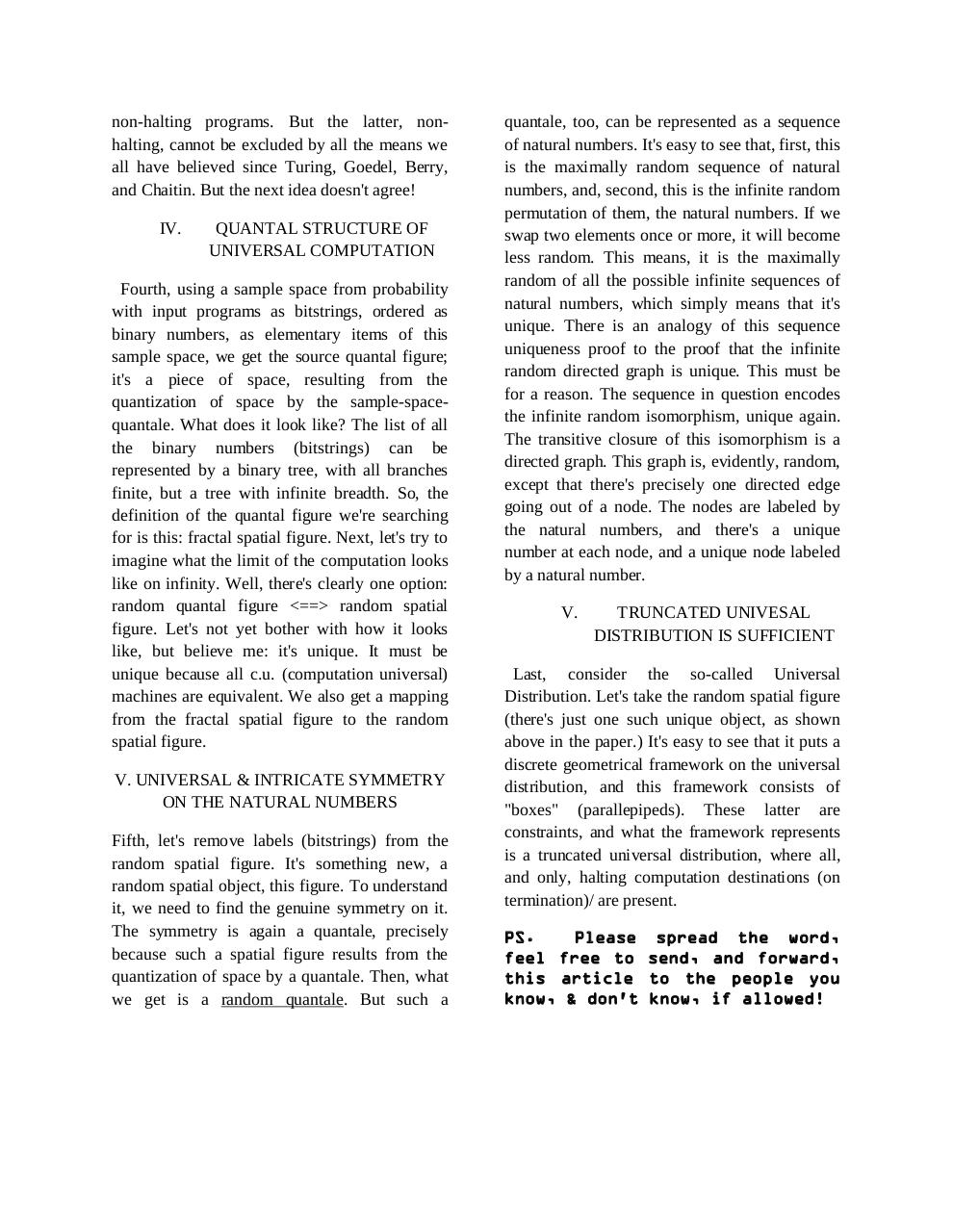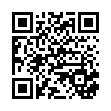# qunatsym .pdf

### File information

Original filename: qunatsym.pdf

This PDF 1.5 document has been generated by MicrosoftÂ® Office Word 2007, and has been sent on pdf-archive.com on 06/09/2015 at 07:50, from IP address 107.135.x.x. The current document download page has been viewed 615 times.
File size: 34 KB (3 pages).
Privacy: public file

qunatsym.pdf (PDF, 34 KB)

### Document preview

The Genuine Symmetry on Natural Numbers:
Truncated Universal Distribution is Enough
Artem S. Shafraniuk
Master of Scientific and Technical Computing; Loyola University Chicago, Illinois, United States of America
Bachelor of Computer Science, Master of Information Control Systems and Technologies; European University, Kiev, Ukraine

(Dated: September 5, 2015)

author's e-mail: artem.shafraniuk@gmail.com

The author analyzed the structure of universal computation, and came to the result that the structure
of its limit on infinity consists of constraints on its results. Then he has found out that the non-halting
programs can be taken out of consideration, by using point-free topology &amp; quantale representation.
At last, for effective purposes, the universal distribution can also be pruned. The main result is that
there is a symmetry on natural numbers, which is their infinite random permutation. This one is
unique: any other sequence can be obtained from the author's one by swapping any two elements of
the sequence one or more times. But this always, and only, decreases the randomness of the sequence
in question. So this sequence is also the maximally random sequence of natural numbers.
hereby the author claims an exclusive discovery of an idea made for the first time and copyright to its formulation

I.

INTRODUCTION TO FORMAL
COMPUTATION

First, let's take a look at formal computation
models from Computer Science. It is known that
some are universal, and the rest are not. A
computation model is universal if it can execute
any algorithm possible. (Examples are Turing
Machine, Random Access Machine, Universal
Recursive Function, Universal mu-Function,
Queue Machine, etc.) Also, it, a machine, has an
input, and an output, and what it does is, it
transforms the input (an algorithm or a program)
into the output, if possible. The reason for the
exceptions is, not all algorithms halt, and this is
the only case in which it is impossible for the
computation model, also called a machine, to
halt, and give an output, in finite time.
Evidently, if the machine doesn't halt in finite
time, it doesn't halt at all, so it cannot provide
any output. It is also known that any
computation universal machine can simulate any
other computation universal machine, using a
program (adaptor) that simulates that other one.

This is clear from the fact that any computation
universal machine can run any algorithm, and
also that any computation universal machine can
be represented by an algorithm. G. Chaitin has
discovered a very curious real number, which he
called Omega. In binary expansion it starts with
zero, and then at each position i (index),
progressing towards the right of the number's
expansion, the bit x_i is '1' if the program
number i halts, and '0' otherwise (if the program
number i doesn't halt.) Now, these programs
represent algorithms, so this is a matter of
coding. Also, since a program is a finite discrete
object, it can be directly encoded by a natural
number. Then, it's clear to see that all Omega's
(for all possible different computation universal
machines) are bit-wise isomorphic, as are
algorithms indexed by the i's] in a Omega's. So,
any computation universal machine takes an
input, runs, and then either halts at a finite step,
and provides an output; or doesn't halt at all. It
runs in steps (sometimes called tacts). It is easy
to see that, for any classical computation
universal machine, any of its states, including
the start state, which is the input itself, can be

represented by a natural number, and the
machine itself then can be presented as an
iterated function o=f(i), where i is input, o is
output. This function then iterates on itself,
starting from the input, until it reaches a limit
cycle, which is a state s=f(s). This s then is taken
to be the output. Such a machine, then, can be
otherwise interpreted being "tabulated" into an
infinite sequence of natural numbers f(0), f(1),
...f(i), ... . Executing a program then means
picking start index s, and looking up what's at
that index, then changing state, s:=f(s). The
author of this paper has published such a
sequence before, based on a pairwise encoding
of the calculus of combinators S &amp; K. Any
combinator possible is then encoded as a natural
number. This sequence was published online, in
the Encyclopedia of Integer Sequences.
Actually, any computation universal machine,
represented by a function, is a homomorphism,
and, represented as a sequence, is a
pseudorandom one. In the limit it (computation /
iteration) converges to a random sequence,
except for the infinities at the positions
corresponding to the non-halting input
programs. Now, if we knew Omega, we could
construct an oracle for the halting problem, and
compute the limit, which latter, again, will come
out to be random.
II.

COMPLEXITY OF UNIVERSAL
COMPUTATION

Second, there was a famous mathematician A.
Kolmogorov, who discovered two brilliant
things that we need to know. The first is the
Axiomatic Probability Theory, and the second is
called Kolmogorov Complexity. From the first
we only need to look at the sample space, which
is the set of all sets of n possible elementary
items, so the number of sets is then 2^n. The
second, Kolmogorov Complexity is written
down KC(o) = min{l(p)} : o=M(p), where KC Kolmogorov Complexity, M - computation
universal machine, p - any program, min{l(p)}:

o=M(p) - the length of the minimal program,
such that if M takes it as input and runs, it's
output is o. Next, let's take a Universal Turing
Machine (UTM) as our model for computation.
One should note, however, that it, a UTM, is a
program on the standard Turing Machine (TM),
being an adaptor, which is also computation
universal. Each of its states, either from input to
the output, or from input to infinity (if it doesn't
halt on that input), is a finite string of bits. Let's
call it a bitstring. Now, observe that if we add a
'0.' on the left to a finite bitstring, we always get
a rational number. Also, all the possible inputs
to our UTM are, then, all the rational numbers,
and so its, UTM's, one-step iteration is a
homomorphism on the rational numbers. Let's
call the UTM with bitstring states the Bitstring
Universal Turing Machine, or BUTM.
III.

QUANTAL TOPOLOGY &amp;
COMPUTATION

Third, let's introduce a concept, from Point
Free Topology, called a quantale, discovered by
Mulvey. A quantale is, by definition, an upperjoin semi-lattice, and its original primary role is
quantization of space, or something spatial.
Next, the sample space from Kolmogorov's
Probability Theory can, in general, be
represented by a quantale, as there is an
isomorphism from the first to the second. The
sample space contains items (usually events,
constructed from elementary events, as their
power set. So the sample space then contains all
the possible combinations of the elementary
events.) Let's call the spatial object that results
from the quantization of space by such a
quantale, a quantal figure or spatial figure, such
that they coincide. It should be constructed,
using point-free topology, from the sample
space, represented by a labeled quantale,
isomorphic to the sample space; with labels
being the elementary items / "events". Now,
bitstring-programs -to- bitstring-results, using
BUTM, *is* a homomorphism, except for the

non-halting programs. But the latter, nonhalting, cannot be excluded by all the means we
all have believed since Turing, Goedel, Berry,
and Chaitin. But the next idea doesn't agree!
IV.

QUANTAL STRUCTURE OF
UNIVERSAL COMPUTATION

Fourth, using a sample space from probability
with input programs as bitstrings, ordered as
binary numbers, as elementary items of this
sample space, we get the source quantal figure;
it's a piece of space, resulting from the
quantization of space by the sample-spacequantale. What does it look like? The list of all
the binary numbers (bitstrings) can be
represented by a binary tree, with all branches
finite, but a tree with infinite breadth. So, the
definition of the quantal figure we're searching
for is this: fractal spatial figure. Next, let's try to
imagine what the limit of the computation looks
like on infinity. Well, there's clearly one option:
random quantal figure &lt;==&gt; random spatial
figure. Let's not yet bother with how it looks
like, but believe me: it's unique. It must be
unique because all c.u. (computation universal)
machines are equivalent. We also get a mapping
from the fractal spatial figure to the random
spatial figure.
V. UNIVERSAL &amp; INTRICATE SYMMETRY
ON THE NATURAL NUMBERS
Fifth, let's remove labels (bitstrings) from the
random spatial figure. It's something new, a
random spatial object, this figure. To understand
it, we need to find the genuine symmetry on it.
The symmetry is again a quantale, precisely
because such a spatial figure results from the
quantization of space by a quantale. Then, what
we get is a random quantale. But such a

quantale, too, can be represented as a sequence
of natural numbers. It's easy to see that, first, this
is the maximally random sequence of natural
numbers, and, second, this is the infinite random
permutation of them, the natural numbers. If we
swap two elements once or more, it will become
less random. This means, it is the maximally
random of all the possible infinite sequences of
natural numbers, which simply means that it's
unique. There is an analogy of this sequence
uniqueness proof to the proof that the infinite
random directed graph is unique. This must be
for a reason. The sequence in question encodes
the infinite random isomorphism, unique again.
The transitive closure of this isomorphism is a
directed graph. This graph is, evidently, random,
except that there's precisely one directed edge
going out of a node. The nodes are labeled by
the natural numbers, and there's a unique
number at each node, and a unique node labeled
by a natural number.
V.

TRUNCATED UNIVESAL
DISTRIBUTION IS SUFFICIENT

Last, consider the so-called Universal
Distribution. Let's take the random spatial figure
(there's just one such unique object, as shown
above in the paper.) It's easy to see that it puts a
discrete geometrical framework on the universal
distribution, and this framework consists of
"boxes" (parallepipeds). These latter are
constraints, and what the framework represents
is a truncated universal distribution, where all,
and only, halting computation destinations (on
termination)/ are present.
PS.
feel free to send, and forward,
know, &amp; don't know, if allowed!#### HTML Code

Copy the following HTML code to share your document on a Website or Blog

#### QR Code### Related keywords